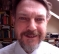## Proof of Correlation Theorem for DFTStarted by 2 years ago4 replieslatest reply 2 years ago145 views

Goodmorning to everyone. I was trying to understand the proof of the cross-correlation theorem for the DFT given in the dsprelated website

It defines the cross-correlation between the x and y sequences as:

$$x\star y = \sum_{m = 0}^{N-1} \overline{x(m)}y(n+m)$$

then the substitution $$m \leftarrow -m$$ is performed, but I don't understand why the result of the substitution is:

$$= \sum_{m = 0}^{N-1} \overline{x(-m)}y(n-m)$$

In fact,  I obtain the following equation, making the substitution $$u \leftarrow -m$$:

$$\sum_{u = 0}^{1-N} \overline{x(-u)}y(n-u) = \sum_{u = 1-N}^{0} \overline{x(-u)}y(n-u)$$

that is different from the previous equation in the summation range.

Can you find where is my mistake?

[ - ]
Reply by June 10, 2020Is this a cyclic convolution? (Probably yes, since this is DSP...) If so, add N to any negative indexes to make them from 0 to N-1. In particular, if m goes from -(N-1) to 0, then this is the same as 1 to N (but N itself is actually 0).

[ - ]
Reply by June 10, 2020Looking at the proof referred too, it contains the note:

"Also, the summation range in the second line is equivalent to the range because all indexing is modulo"

[ - ]
Reply by June 10, 2020split u=1-N= -(N-1) in to two parts. -(N-1) to -1 and 0.Here everything is modulo N.So -(N-1) to -1 corresponds 1 to N-1.Added with 0 it gives 0 to N-1.This is because for -ve index of x and y correspond to N added to make it +Ve due to modulo operation

[ - ]
Reply by June 10, 2020Thanks  to everybody.

I needed a bit of time to visualize it but finally I did it.

I post here a verbose proof, hoping that it can be useful.

$$z[\tau]$$ is defined as the circular cross-correlation function between x[m] and y[m], as follows:

$$z[\tau] = x[m]\otimes y[m] =\sum_{m=0}^{N-1}x^*[(m)_{mod N}]y[(\tau+m)_{mod N}]$$

It has to be observed that $$z[\tau]$$ is a sequence where each element correspond to the cross-correlation value for the displacement tau. All the indexing is handled by the modulo N operation so that the finite-length correlating sequence is accessed circularly.

Now the substitution $$u = -m$$ is performed:

$$x[m]\otimes y[m] = \sum_{u=-(N-1)}^{0}x^*[(-u)_{mod N}]y[(\tau-u)_{mod N}]$$

The range of the summation is $$\left\lbrace -(N-1),-(N-2),..,-1,0\right\rbrace$$. For example the index -1 correspond to the index N-1 when a sequence is circularly accessed. So a factor N has to be added to all of the negative indexes.  This means that the index u goes from 0 to N-1, like the index m.

So the equation can be written as follows:

$$x[m]\otimes y[m] =\sum_{m=0}^{N-1}x^*[(-m)_{mod N}]y[(\tau-m)_{mod N}]$$